# Interesting Charge Separation Problem

Here is a problem sent by Neeraj.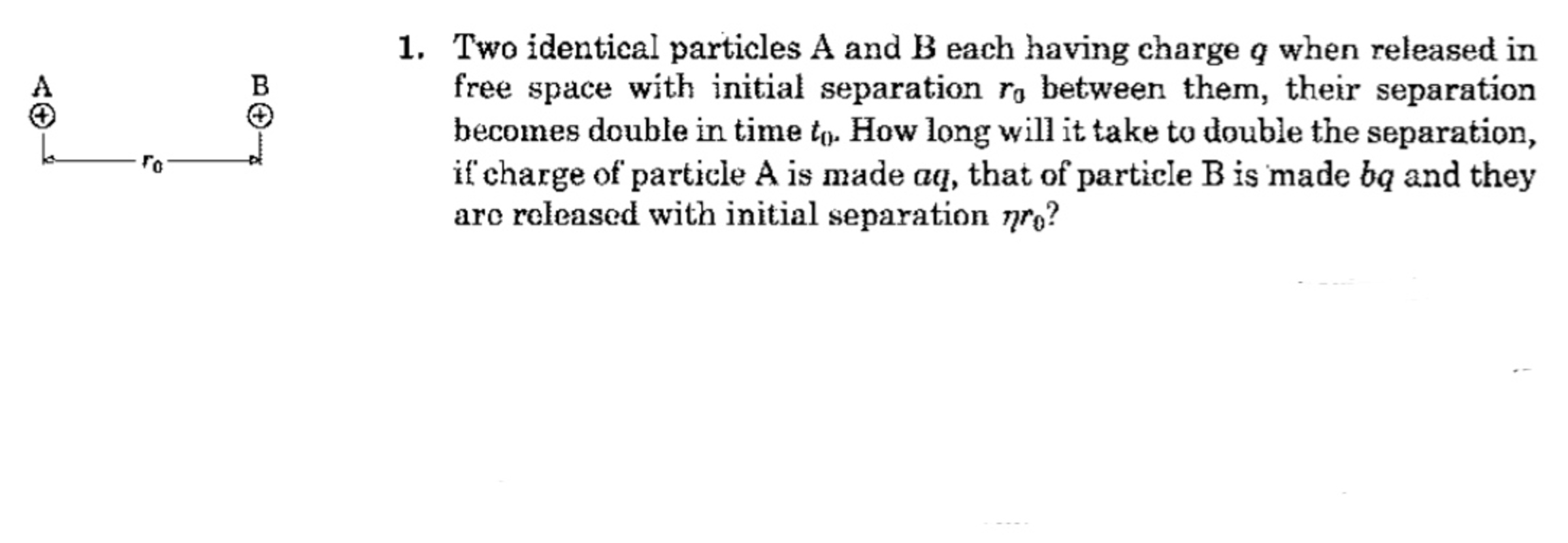Let $x = 0$ be the vertical line right in between the particles. Both particles have the same mass and experience the same force magnitude, so they will be equidistant from $x = 0$ at all times. Let $x$ be the distance of each particle away from the axis. The differential equation for this system is:

$\frac{k q^2}{(2x)^2} = \frac{k q^2}{4x^2} = m \ddot{x}$

This differential equation seems pretty nasty. I looked around online for analytical solutions and didn't find much. Nevertheless, the simulation is very easy to run numerically. The strategy is to run the base case first and call the final time $t_0$. Then run again with parameters $a, b , \eta$ changed from their original values, and see how the final time changes.

Multiplying the charge magnitudes by $a$ and $b$ causes the final time to be multiplied by $\frac{1}{\sqrt{a b}}$. Multiplying the initial separation by $\eta$ causes the final time to be multiplied by $\sqrt{\eta^3}$. So the final result is:

$t_1 = t_0 \sqrt{\frac{\eta^3}{a b}}$

Simulation code is attached:

  1 2 3 4 5 6 7 8 9 10 11 12 13 14 15 16 17 18 19 20 21 22 23 24 25 26 27 28 29 30 31 32 33 34 35 36 37 38 39 40 41 42 43 44 45 46 47 48 49 50 51 52 53 54 55 56 57 58 59 60 61 62 63 import math dt = 10.0**(-5.0) m = 1.0 k = 1.0 q = 1.0 x0 = 1.0 ########################### # Base case t = 0.0 x = x0 xd = 0.0 xdd = 0.0 while x <= 2.0*x0: x = x + xd*dt xd = xd + xdd*dt F = k*q*q/((2.0*x)**2.0) xdd = F/m t = t + dt t0 = t ########################### # Modified case a = 1.0 b = 1.0 n = 1.0 t = 0.0 x = n*x0 xd = 0.0 xdd = 0.0 while x <= 2.0*n*x0: x = x + xd*dt xd = xd + xdd*dt F = k*(a*q)*(b*q)/((2.0*x)**2.0) xdd = F/m t = t + dt t1 = t ########################### print dt print (t1/t0)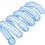Note by Steven Chase
11 months, 1 week ago

This discussion board is a place to discuss our Daily Challenges and the math and science related to those challenges. Explanations are more than just a solution — they should explain the steps and thinking strategies that you used to obtain the solution. Comments should further the discussion of math and science.

When posting on Brilliant:

• Use the emojis to react to an explanation, whether you're congratulating a job well done , or just really confused .
• Ask specific questions about the challenge or the steps in somebody's explanation. Well-posed questions can add a lot to the discussion, but posting "I don't understand!" doesn't help anyone.
• Try to contribute something new to the discussion, whether it is an extension, generalization or other idea related to the challenge.

MarkdownAppears as
*italics* or _italics_ italics
**bold** or __bold__ bold
- bulleted- list
• bulleted
• list
1. numbered2. list
1. numbered
2. list
Note: you must add a full line of space before and after lists for them to show up correctly
paragraph 1paragraph 2

paragraph 1

paragraph 2

[example link](https://brilliant.org)example link
> This is a quote
This is a quote
    # I indented these lines
# 4 spaces, and now they show
# up as a code block.

print "hello world"
# I indented these lines
# 4 spaces, and now they show
# up as a code block.

print "hello world"
MathAppears as
Remember to wrap math in $$ ... $$ or $ ... $ to ensure proper formatting.
2 \times 3 $2 \times 3$
2^{34} $2^{34}$
a_{i-1} $a_{i-1}$
\frac{2}{3} $\frac{2}{3}$
\sqrt{2} $\sqrt{2}$
\sum_{i=1}^3 $\sum_{i=1}^3$
\sin \theta $\sin \theta$
\boxed{123} $\boxed{123}$

Sort by:

@Steven Chase Thanks I am very grateful to you.

- 11 months, 1 week ago

I was solving this with anayltical method.
Will you take a look in my rough work/attempt???

- 11 months, 1 week ago

Sure, I'm curious to see what you did

- 11 months, 1 week ago

@Steven Chase Nowadays I am studying mathematics only, therefore I am little bit not connected with physics, so I am not able to how to do this problem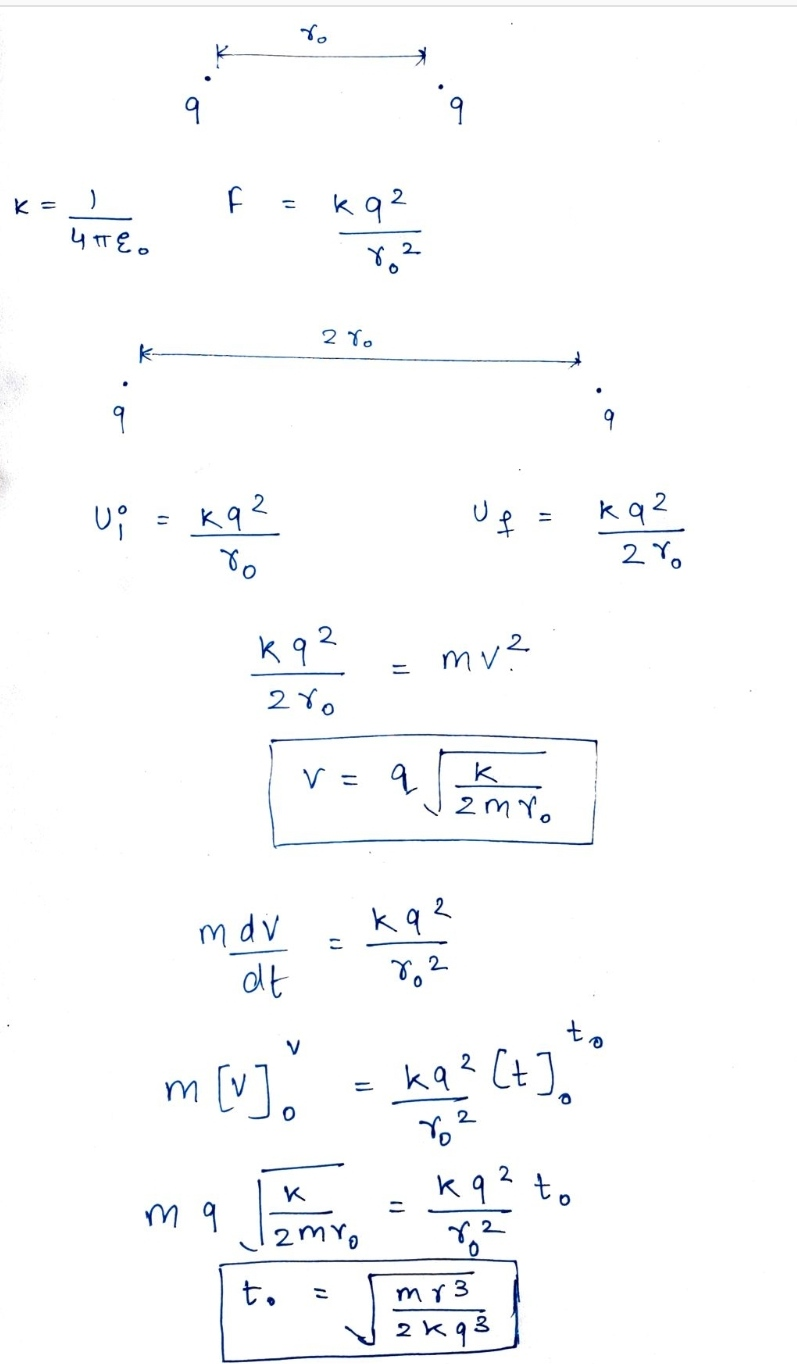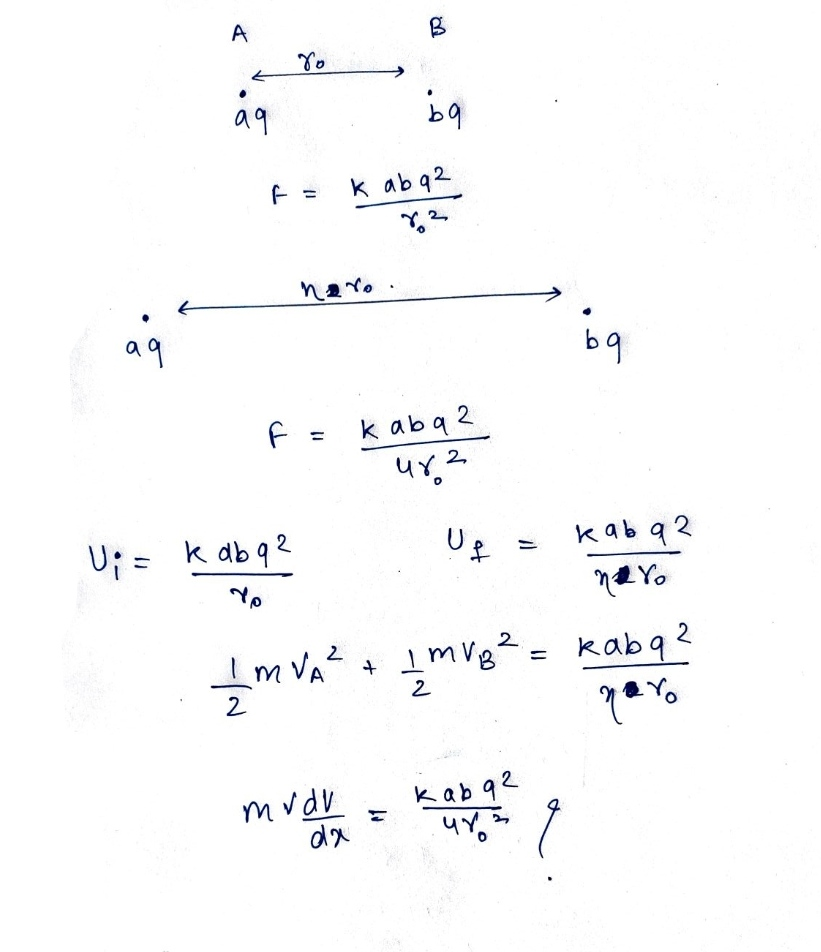- 11 months, 1 week ago

Nice method. I was about to use the hand differential equation solving method posted by Flammable Maths:

And thereby create an equation for the time taken. After all, the nature of gravitational and electrostatic force is very similar; essentially we are solving the same differential equation, so we can use the same method:

$\displaystyle \ddot{r} = \pm \frac{C}{r^2}$

It is a hard differential equation to solve analytically, since it is a nonlinear second order ODE.

- 11 months, 1 week ago

@Steven Chase the above comment is edited.

- 11 months, 1 week ago

Take a look at this video @Lil Doug

The video above shows how much time it takes for two gravitating bodies to collide. Essentially, the differential equation that is to be solved is the same; an inverse square non-linear ODE.

You can apply this to electrostatics as well, despite the fact that the video is about gravity.

- 11 months, 1 week ago

@Steven Chase I think I know how to solve the non-linear ODE above; I sent a video to Neeraj. Here's the video:

It's by Flammable Maths (Jens Genau) who solves a similar ODE above (gravitation). I think the same idea can be applied to electrostatics. So there is a way to do it analytically, I think.

- 11 months, 1 week ago

@Krishna Karthik I am damn sure I can explain better than that guy.

- 11 months, 1 week ago

Flammable maths is an absolutely epic mathematician. He's covered some really interesting videos.

- 11 months, 1 week ago

Thanks for sending that. Looks like a very involved process

- 11 months, 1 week ago

@Steven Chase Hey sir see my method above.
Around 1 year ago i have solved this types of problems that how much time it will take to collide two particle but I don't no why I am not able to do it now .
See my solution above
In the first case, I think my process correct.

- 11 months, 1 week ago

@Steven Chase I think you are the only solver my problem?

- 11 months, 1 week ago

Maybe I could have solved it using a numerical method, but yes, he still his the only solver. Nicely done, too.

Of course, we both know that Steven Chase taught me how to do a time-domain simulation. In retrospect, he, and no one else, taught me how to do a physics simulation. And I'm obviously still not as experienced as him in that (or for that matter, anything in physics).

So, there's a gem of knowledge I think I learnt from him. I mostly looked at his code and learnt how to do it by looking at his code.

In terms of pure programming or coding, I learnt from my father. So, thanks, Steven Chase, for teaching me how to do a time-domain simulation. Cheers.

- 11 months, 1 week ago

@Krishna Karthik I am not saying for this problem bro.
I have posted a another problem in E and M section, I am talking about that.

- 11 months, 1 week ago

Oh, ok. Lol.

- 11 months, 1 week ago

Yeah; that looks mortifyingly hard.

- 11 months, 1 week ago

It seems you have solved all problems.
Do you want more problem?

- 11 months, 1 week ago

Yeah; gimme a Newtonian mechanics problem.

- 11 months, 1 week ago

@Krishna Karthik The problems are taken from my physics book.
There are total 36 problems , Part A contains 33 and Part B contains 3 problems.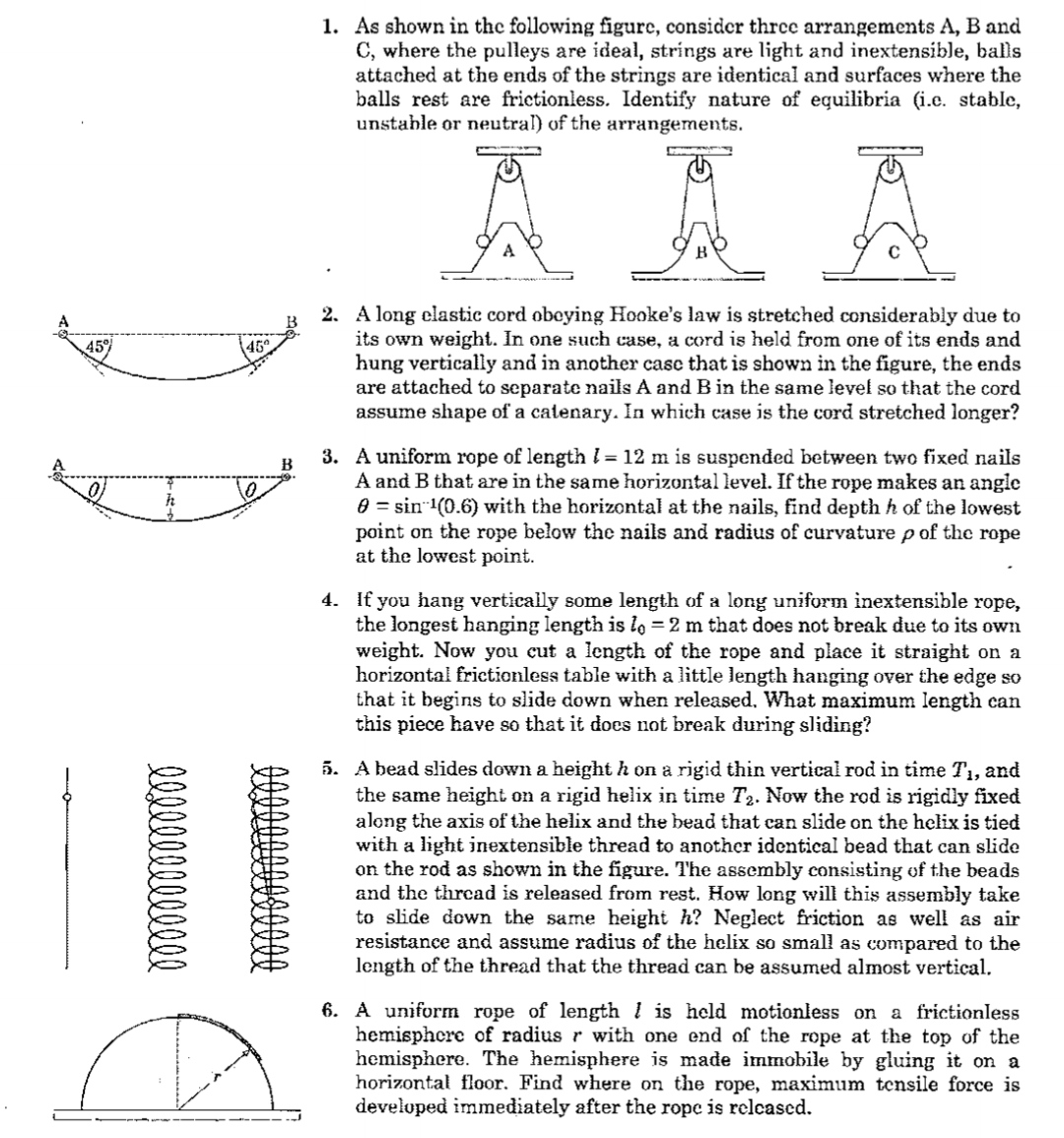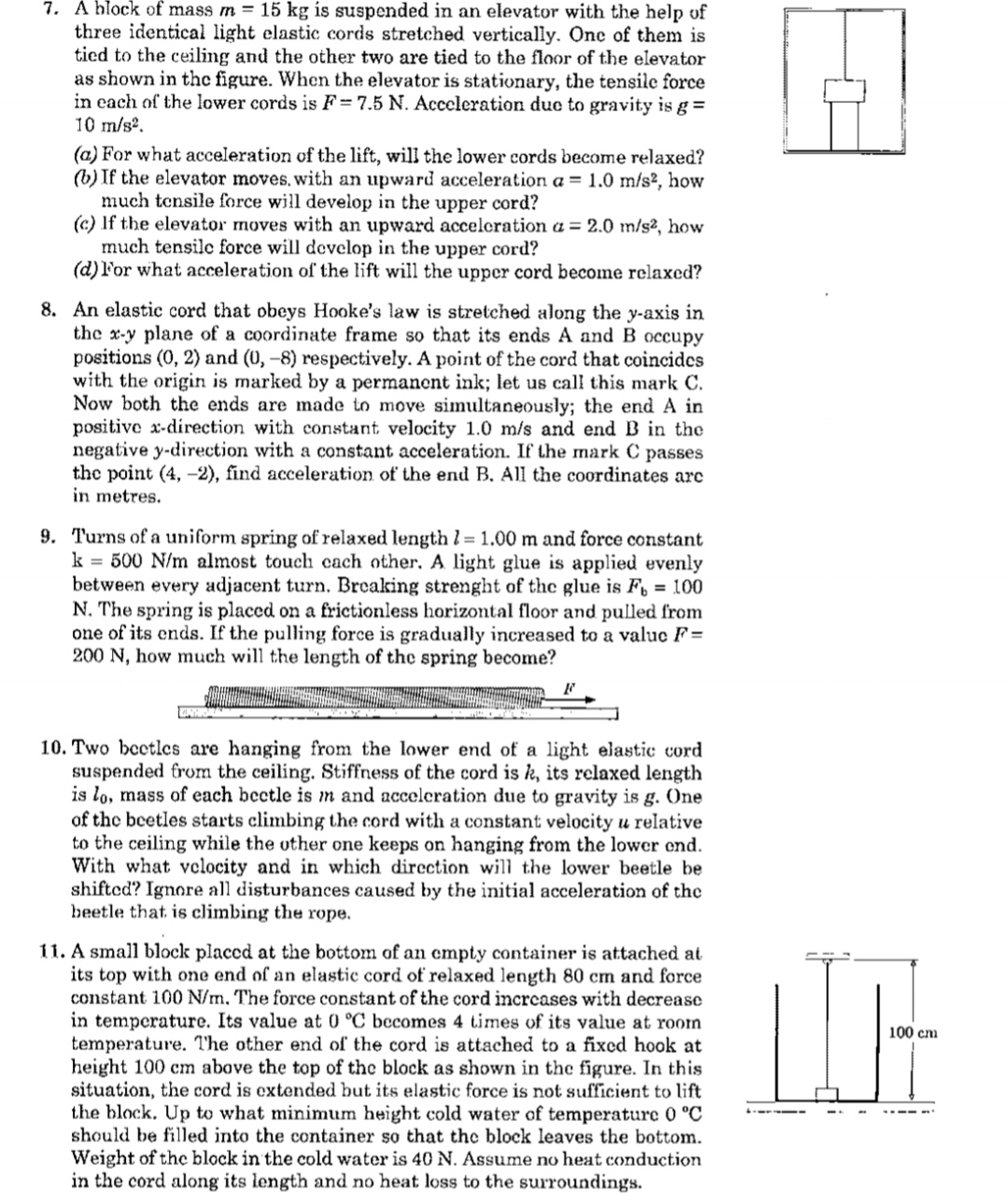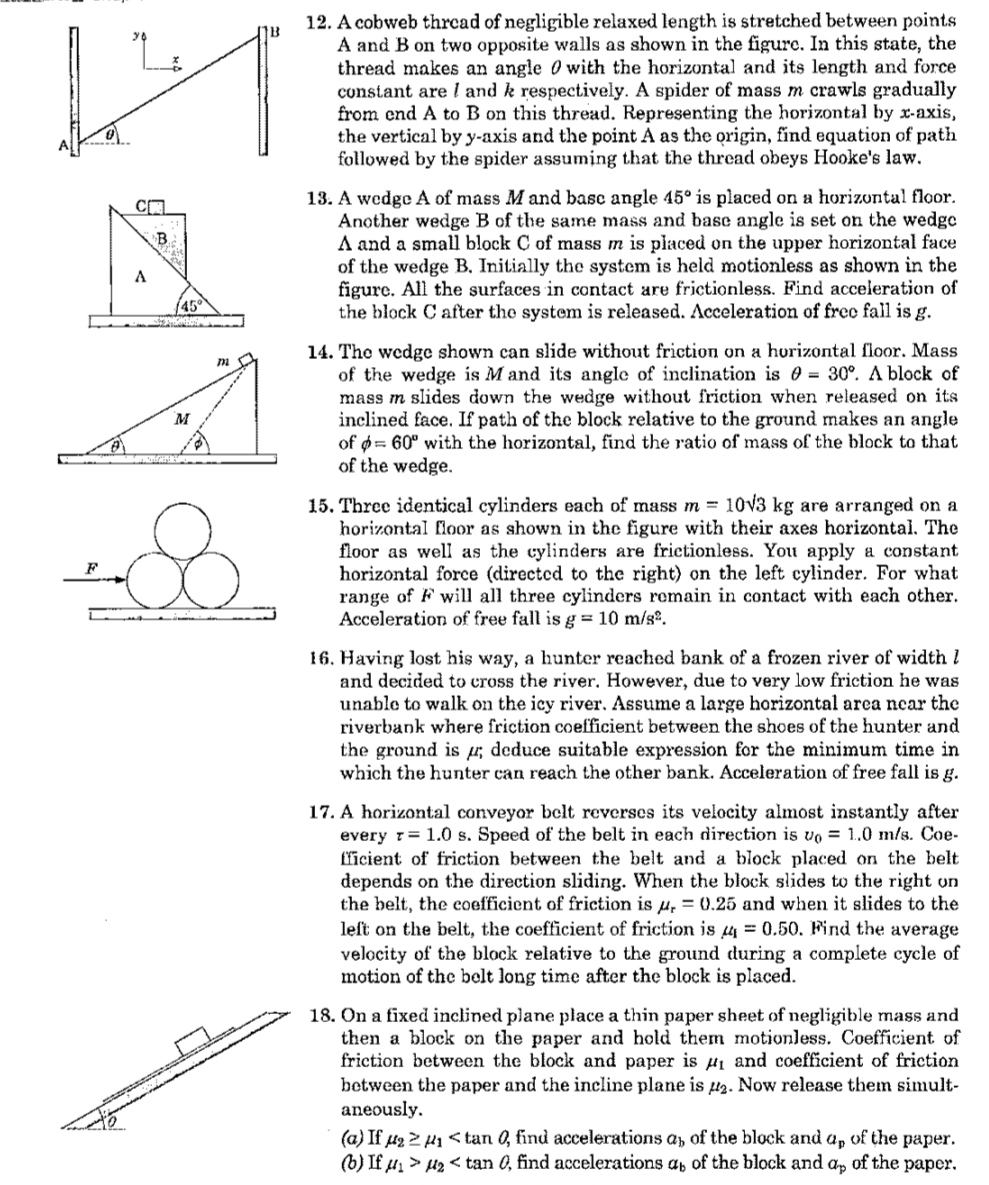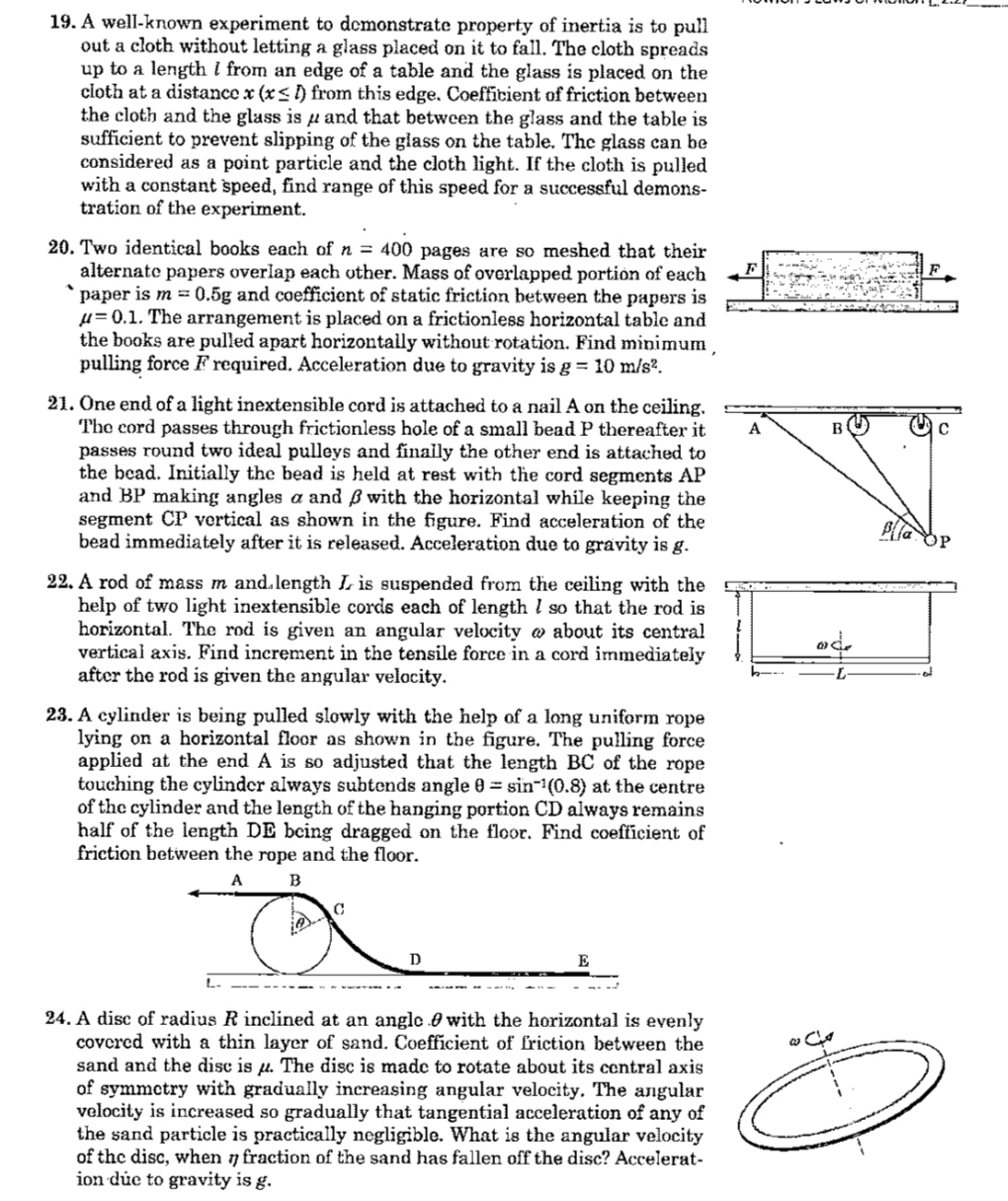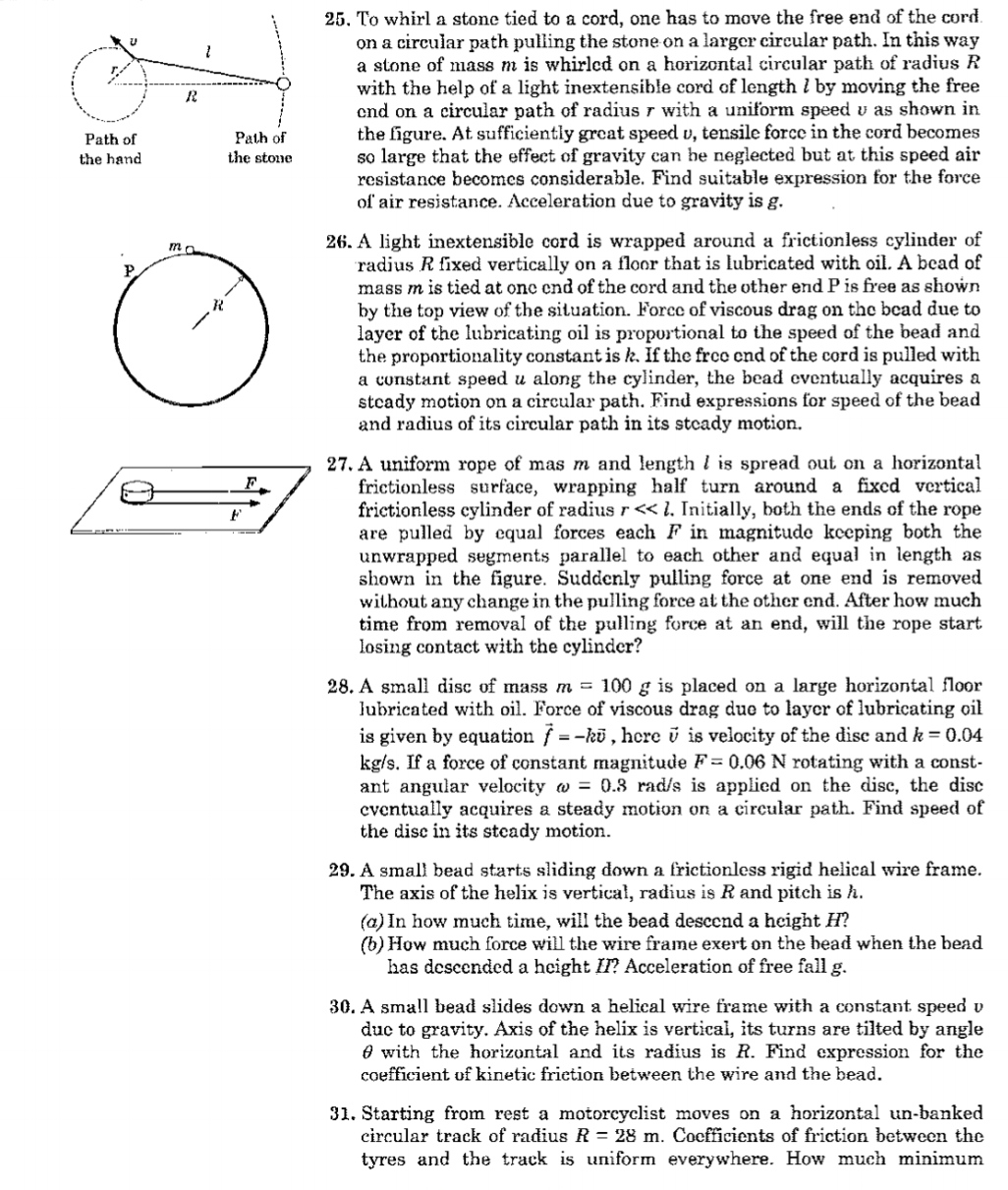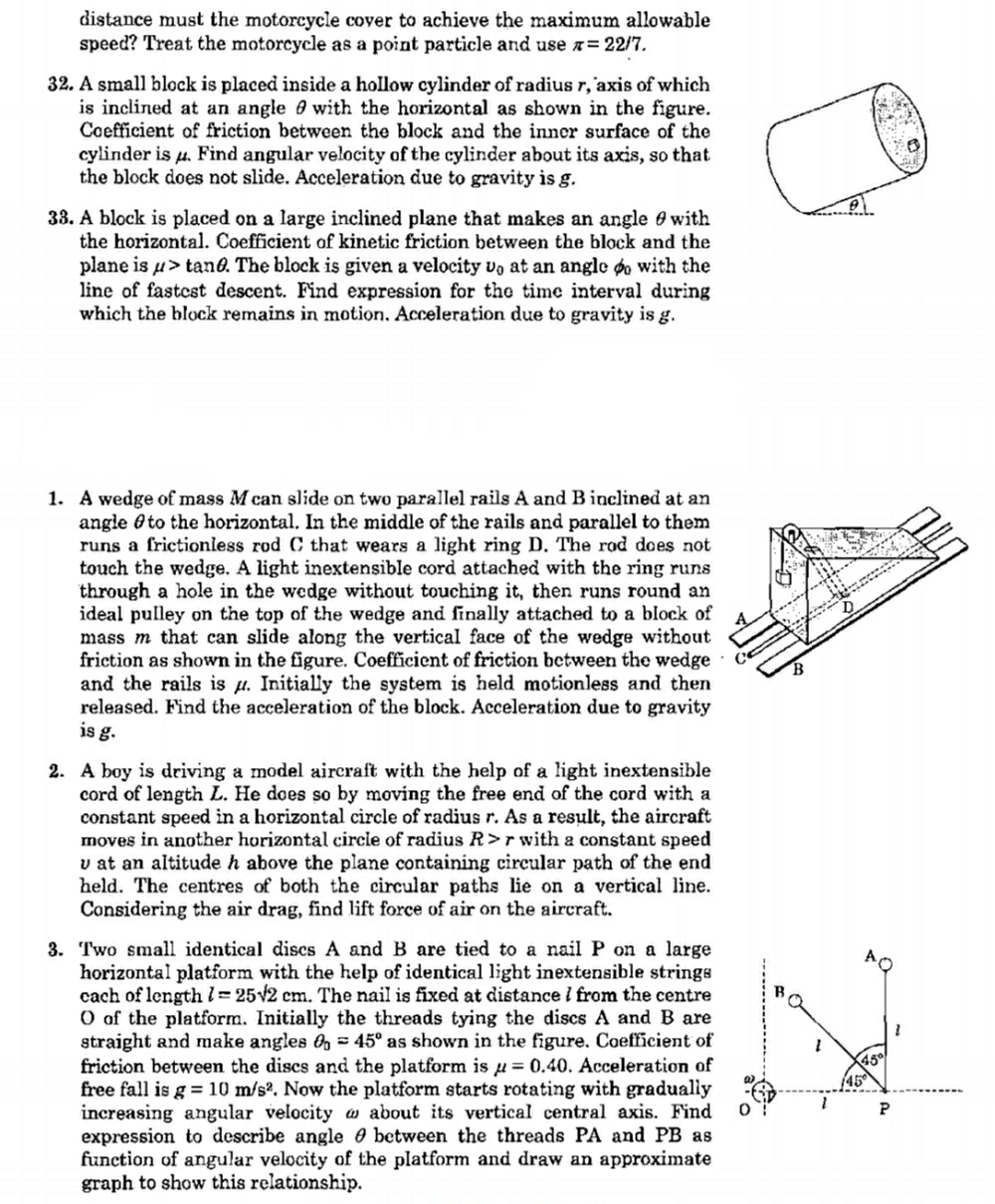- 11 months, 1 week ago

Yo I need some help😫

I'm not able to do no 6.

Pls help.

- 10 months, 3 weeks ago

@Krishna Karthik I have posted a note.

- 10 months, 3 weeks ago

@Steven Chase I have a good attempt for the above problem now.
Would you like to see that?

- 10 months, 3 weeks ago